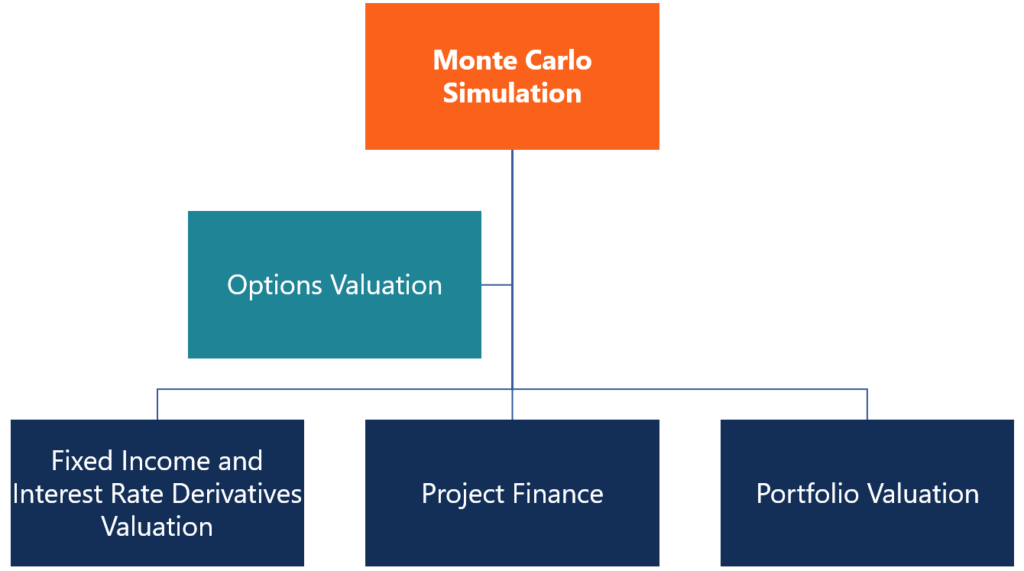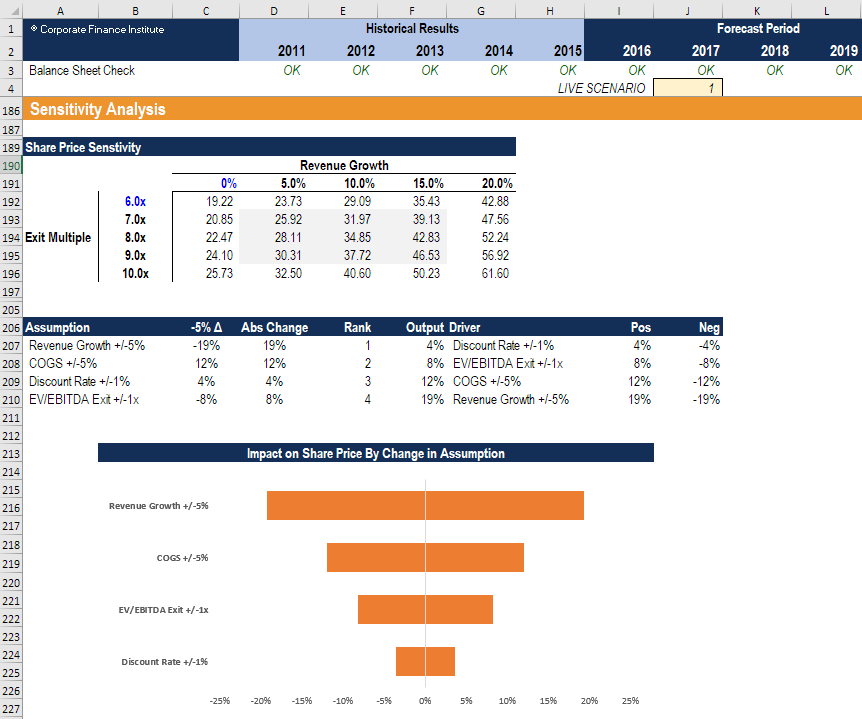# Monte Carlo Simulation

A statistical method applied in modeling the probability of different outcomes in a problem

## What is Monte Carlo Simulation?

Monte Carlo Simulation is a statistical method applied in financial modeling where the probability of different outcomes in a problem cannot be simply solved due to the interference of a random variable. The simulation relies on the repetition of random samples to achieve numerical results. It can be used to understand the effect of uncertainty and randomness in forecasting models.Monte Carlo simulation was first developed by Stanislaw Ulam in the 1940s. Ulam was a mathematician who worked on the Manhattan Project. Initially, the method was derived to solve the problem of determining the average distance neutrons would travel through various materials. The method was named after the Monte Carlo Casino in Monaco since the randomness of the outcomes that is crucial to games such as roulette or dices is essential for Monte Carlo simulations.

In essence, Monte Carlo simulation can be used in almost any probabilistic problem. It explains why it can be used in different fields, including statistics, finance, engineering, and science.

### Theory of Monte Carlo Simulation

The main ideas behind the Monte Carlo simulation are the repeated random sampling of inputs of the random variable and the aggregation of the results. The variable with a probabilistic nature is assigned a random value. The model is then calculated based on the random value. The result of the model is recorded, and the process is repeated. Usually, the process is repeated hundreds or thousand times. When the simulation is complete, the results can be averaged to determine the estimated value.

### Application in Finance

Monte Carlo simulation offers numerous applications in finance. The most common application of the model in finance include:

#### Valuation of options

Monto Carlo simulation is commonly used in equity options pricing. The prices of an underlying share are simulated for each possible price path and the option payoffs are determined for each path. The payoffs are then averaged and discounted to today, which provides the current value of an option. While Monte Carlo simulation works great for European-style options, it is harder to apply the model to value American options.

#### Portfolio valuation

The factors influencing the value of portfolios are simulated, and the portfolio value is calculated. Then, the average value of all simulated portfolios is determined, and the portfolio value is observed.#### Valuation of fixed income instruments and interest rate derivatives

The main source of uncertainty for fixed income instruments and interest rate derivatives is the short rate. The short rate is simulated numerous times, and the price of bond or derivative is determined for each simulated rate. Then, the obtained rates are averaged, and the current value of a bond is determined using this rate.

Project finance and real options analysis: Monte Carlo simulation allows financial analysts constructing stochastic models to assess the project’s Net Present Value (NPV).

#### Financial modeling

When performing sensitivity analysis in financial modeling, it can be Monte Carlo Simulation in Excel. The analysis is performed to test the impact on the net present value (NPV) of the business as underlying assumptions and variables change.Image: CFI’s Sensitivity Analysis Course